# Search by Topic

#### Resources tagged with Odd and even numbers similar to Lots of Biscuits!:

Filter by: Content type:
Age range:
Challenge level:

### There are 64 results

Broad Topics > Numbers and the Number System > Odd and even numbers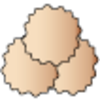### Lots of Biscuits!

##### Age 5 to 7 Challenge Level:

Help share out the biscuits the children have made.### Number Round Up

##### Age 5 to 7 Challenge Level:

Arrange the numbers 1 to 6 in each set of circles below. The sum of each side of the triangle should equal the number in its centre.### The Set of Numbers

##### Age 5 to 7 Challenge Level:

Can you place the numbers from 1 to 10 in the grid?### I Like ...

##### Age 5 to 7 Challenge Level:

Mr Gilderdale is playing a game with his class. What rule might he have chosen? How would you test your idea?### Multiplication Series: Number Arrays

##### Age 5 to 11

This article for teachers describes how number arrays can be a useful reprentation for many number concepts.### Share Bears

##### Age 5 to 7 Challenge Level:

Yasmin and Zach have some bears to share. Which numbers of bears can they share so that there are none left over?### Sorting Numbers

##### Age 5 to 7 Challenge Level:

Can you sort numbers into sets? Can you give each set a name?### Numbers as Shapes

##### Age 5 to 7 Challenge Level:

Use cubes to continue making the numbers from 7 to 20. Are they sticks, rectangles or squares?### Grouping Goodies

##### Age 5 to 7 Challenge Level:

Pat counts her sweets in different groups and both times she has some left over. How many sweets could she have had?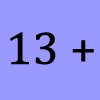### What Could it Be?

##### Age 5 to 7 Challenge Level:

In this calculation, the box represents a missing digit. What could the digit be? What would the solution be in each case?### What Number?

##### Age 5 to 7 Short Challenge Level:

I am less than 25. My ones digit is twice my tens digit. My digits add up to an even number.### How Odd

##### Age 5 to 7 Challenge Level:

This problem challenges you to find out how many odd numbers there are between pairs of numbers. Can you find a pair of numbers that has four odds between them?### Crossings

##### Age 7 to 11 Challenge Level:

In this problem we are looking at sets of parallel sticks that cross each other. What is the least number of crossings you can make? And the greatest?### Becky's Number Plumber

##### Age 7 to 11 Challenge Level:

Becky created a number plumber which multiplies by 5 and subtracts 4. What do you notice about the numbers that it produces? Can you explain your findings?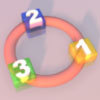### More Numbers in the Ring

##### Age 5 to 7 Challenge Level:

If there are 3 squares in the ring, can you place three different numbers in them so that their differences are odd? Try with different numbers of squares around the ring. What do you notice?### Cube Bricks and Daisy Chains

##### Age 5 to 7 Challenge Level:

Daisy and Akram were making number patterns. Daisy was using beads that looked like flowers and Akram was using cube bricks. First they were counting in twos.### Always, Sometimes or Never?

##### Age 5 to 11 Challenge Level:

Are these statements relating to odd and even numbers always true, sometimes true or never true?### Arrangements

##### Age 7 to 11 Challenge Level:

Is it possible to place 2 counters on the 3 by 3 grid so that there is an even number of counters in every row and every column? How about if you have 3 counters or 4 counters or....?### The Thousands Game

##### Age 7 to 11 Challenge Level:

Each child in Class 3 took four numbers out of the bag. Who had made the highest even number?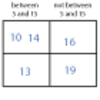### More Carroll Diagrams

##### Age 7 to 11 Challenge Level:

How have the numbers been placed in this Carroll diagram? Which labels would you put on each row and column?### Domino Sorting

##### Age 5 to 7 Challenge Level:

Try grouping the dominoes in the ways described. Are there any left over each time? Can you explain why?### Carroll Diagrams

##### Age 5 to 11 Challenge Level:

Use the interactivities to fill in these Carroll diagrams. How do you know where to place the numbers?### Number Tracks

##### Age 7 to 11 Challenge Level:

Ben’s class were cutting up number tracks. First they cut them into twos and added up the numbers on each piece. What patterns could they see?### Part the Piles

##### Age 7 to 11 Challenge Level:

Try to stop your opponent from being able to split the piles of counters into unequal numbers. Can you find a strategy?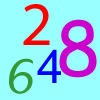### Largest Even

##### Age 5 to 7 Challenge Level:

How would you create the largest possible two-digit even number from the digit I've given you and one of your choice?### One of Thirty-six

##### Age 5 to 7 Challenge Level:

Can you find the chosen number from the grid using the clues?### Pairs of Legs

##### Age 5 to 7 Challenge Level:

How many legs do each of these creatures have? How many pairs is that?### Square Subtraction

##### Age 7 to 11 Challenge Level:

Look at what happens when you take a number, square it and subtract your answer. What kind of number do you get? Can you prove it?### Two Numbers Under the Microscope

##### Age 5 to 7 Challenge Level:

This investigates one particular property of number by looking closely at an example of adding two odd numbers together.### Odds and Threes

##### Age 7 to 11 Challenge Level:

A game for 2 people using a pack of cards Turn over 2 cards and try to make an odd number or a multiple of 3.### Odd Times Even

##### Age 5 to 7 Challenge Level:

This problem looks at how one example of your choice can show something about the general structure of multiplication.### What Do You Need?

##### Age 7 to 11 Challenge Level:

Four of these clues are needed to find the chosen number on this grid and four are true but do nothing to help in finding the number. Can you sort out the clues and find the number?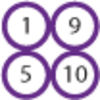### Pairs of Numbers

##### Age 5 to 7 Challenge Level:

If you have ten counters numbered 1 to 10, how many can you put into pairs that add to 10? Which ones do you have to leave out? Why?### Curious Number

##### Age 7 to 11 Challenge Level:

Can you order the digits from 1-3 to make a number which is divisible by 3 so when the last digit is removed it becomes a 2-figure number divisible by 2, and so on?### Magic Vs

##### Age 7 to 11 Challenge Level:

Can you put the numbers 1-5 in the V shape so that both 'arms' have the same total?### Play to 37

##### Age 7 to 11 Challenge Level:

In this game for two players, the idea is to take it in turns to choose 1, 3, 5 or 7. The winner is the first to make the total 37.### Always, Sometimes or Never? Number

##### Age 7 to 11 Challenge Level:

Are these statements always true, sometimes true or never true?### Even and Odd

##### Age 5 to 7 Challenge Level:

This activity is best done with a whole class or in a large group. Can you match the cards? What happens when you add pairs of the numbers together?### Ring a Ring of Numbers

##### Age 5 to 7 Challenge Level:

Choose four of the numbers from 1 to 9 to put in the squares so that the differences between joined squares are odd.### Three Spinners

##### Age 7 to 11 Challenge Level:

These red, yellow and blue spinners were each spun 45 times in total. Can you work out which numbers are on each spinner?### Odd Squares

##### Age 7 to 11 Challenge Level:

Think of a number, square it and subtract your starting number. Is the number you’re left with odd or even? How do the images help to explain this?### Venn Diagrams

##### Age 5 to 11 Challenge Level:

Use the interactivities to complete these Venn diagrams.### Sets of Four Numbers

##### Age 7 to 11 Challenge Level:

There are ten children in Becky's group. Can you find a set of numbers for each of them? Are there any other sets?### Sets of Numbers

##### Age 7 to 11 Challenge Level:

How many different sets of numbers with at least four members can you find in the numbers in this box?### Down to Nothing

##### Age 7 to 11 Challenge Level:

A game for 2 or more people. Starting with 100, subratct a number from 1 to 9 from the total. You score for making an odd number, a number ending in 0 or a multiple of 6.### Seven Flipped

##### Age 7 to 11 Challenge Level:

Investigate the smallest number of moves it takes to turn these mats upside-down if you can only turn exactly three at a time.### A Mixed-up Clock

##### Age 7 to 11 Challenge Level:

There is a clock-face where the numbers have become all mixed up. Can you find out where all the numbers have got to from these ten statements?### Next-door Numbers

##### Age 5 to 7 Challenge Level:

Florence, Ethan and Alma have each added together two 'next-door' numbers. What is the same about their answers?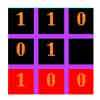### Odd Tic Tac

##### Age 5 to 7 Challenge Level:

An odd version of tic tac toe### Number Differences

##### Age 7 to 11 Challenge Level:

Place the numbers from 1 to 9 in the squares below so that the difference between joined squares is odd. How many different ways can you do this?